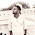# What is the NumPy Library | Python NumPy | Part-1 | Tipszon# Introduction to Python NumPy Library

• NumPy is the fundamental package for scientific computing in Python. It is a Python library that provides a multidimensional array object, various derived objects (such as masked arrays and matrices),
• and an assortment of routines for fast operations on arrays, including mathematical, logical, shape manipulation, sorting, selecting, I/O, discrete Fourier transforms, basic linear algebra, basic statistical operations, random simulation and much more.

## Numeric :

• The ancestor of NumPy, was developed by Jim Hugunin. Another package Numarray was also developed, having some additional functionalities.
•  In 2005, Travis Oliphant created NumPy package by incorporating the features of Numarray into Numeric package. There are many contributors to this open source project.

## NumPy written In Python and C

• Mathematical algorithms written for this version of Python often run much slower than compiled equivalents.
• NumPy addresses the slowness problem partly by providing multidimensional arrays and functions and operators that operate efficiently on arrays, requiring rewriting some code, mostly inner loops, using NumPy.

## Why is NumPy Fast?

Vectorization describes the absence of any explicit looping, indexing, etc., in the code - these things are taking place, of course, just “behind the scenes” in optimized, pre-compiled C code. Vectorized code has many advantages, among which are:

•  vectorized code is more concise and easier to read
• fewer lines of code generally means fewer bugs
• the code more closely resembles standard mathematical notation (making it easier, typically, to correctly code mathematical constructs)
• vectorization results in more “Pythonic” code. Without vectorization, our code would be littered with inefficient and difficult to read for loops.

1.1.